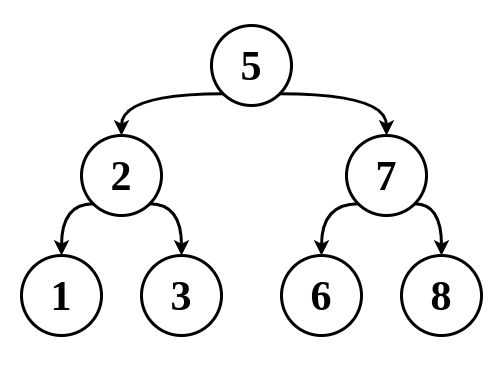# Ricardo Borges

Personal blog## How to check if a Binary Tree is a BST

This is one of those problems that have properties that, given an example, we can identify them right away, like "check if this array is ordered", if it contains only a few elements we just have to look at it and we will know.

Before we continue, if necessary, take some time to understand what is a BST reading this post.

For example, look at this Binary tree:You know this is a BST because it satisfy the definition: `left.data <= current.data < right.data`.

So far, we understand the problem and we can identify a BST just looking at it, what is pending now, is the algorithm. Basically, all we have to do is check if every subtree satisfies the definition of the BST, let's see how we would do that.

• Starting with the root node, well that node can have any value, in this case, 5;
• Moving on to the left child node 2, now this node can't be greater than its parent (5);
• Node 2's left child 1 also has a limit (2);
• Node 2's right child 3 must have a value that is greater than its parent (2) but less than 5.

Up until now, we have noticed that some node has to be between a minimum and maximum value. Left nodes don't have a minimum constraint, and the root node doesn't have any constraints at all.

• Now moving to the right subtree, the root node's right child 7, has to be greater than its parent;
• Node 7's right child 8 must be greater than 7;
• Node 7's left child 6 must be between 5 and 7.

Now we know that both subtrees have similar constraints, with this information, we know that our algorithm will start with the root node and will check if some nodes are between some min and max values, while other nodes don't have a min or max limit:

``1function isBST(node: BinarySearchTreeNode<number>, min: number, max: number) {}``

We can do this recursively, so we need a base case:

``````1function isBST(node: BinarySearchTreeNode<number>, min: number, max: number) {
2  if (!node) return true; // base case
3}
``````

Next step is to check if the current node, is between those min and max values, we also have to consider that some nodes don't have those constraints, so we can expect that min and max can be null:

``````1function isBST(node: BinarySearchTreeNode<number>, min: number, max: number) {
2  if (!node) return true; // base case
3
4  if ((min && node.data <= min) || (max && node.data > max)) {
5    return false;
6  }
7}
``````

Finally, we have to do the same for left and right subtrees, if any of them don't satisfy the BST definition we simply return false

``````1function isBST(node: BinarySearchTreeNode<number>, min: number, max: number) {
2  if (!node) return true; // base case
3
4  if ((min && node.data <= min) || (max && node.data > max)) {
5    return false;
6  }
7
8  // if the right or the left subtree is not a BST, return false
9  if (
10    !isBST(node.leftNode, min, node.data) ||
11    !isBST(node.rightNode, node.data, max)
12  ) {
13    return false;
14  }
15
16  // finally, if all subtrees are under those constraints, return true
17  return true;
18}
``````

That's it, I tried to explain step by step this algorithm, I hope I succeed.